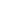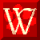Superior Compressed Natural Gas Dispensers, Parts, & ServiceHow Natural Gas is Measured

Natural Gas is usually measured by volume and is stated in cubic feet. A cubic foot of gas is the amount of gas needed to fill a volume of one cubic foot under set conditions of pressure and temperature.To measure larger amounts of natural gas, a "therm" is used to denote 100 cubic feet, and "mcf" is used to denote 1,000 cubic feet.

To provide greater accuracy in comparing fuels, energy content is measured in terms of "British Thermal Units (BTU's)." A BTU is the amount of heat required to raise one pound of water (approximately a pint), one degree Farenheit at or close to its point of maximum density.

The energy content of natural gas differs in various locations thoughout the country.  For the sake of comparison, one average cubic foot of natural gas about 1,000 BTU's of heat energy.The chart below shows how much heat energy is released in various quantities of natural gas.

HEAT ENERGY PER UNIT OF MEASURE FOR NATURAL GAS

 UNIT OF MEASURE APPROX. HEAT ENERGY 1 cubic foot 1,000 BTU's 100 cubic feet (1 therm) 100,000 BTU's 1,000 cubic feet (1 mcf) 1,000,000 BTU's

How Natural Gas is Sold as Transportation Fuel

Compressed Natural Gas or CNG is sold at the retail level either by mass, energy units or "gasoline gallon equivalents" or gge. The National Conference of Weights & Measurements (NCWM) has developed a standard unit of measurement for compressed natural gas. The standard is defined in the NIST Handbook 44 Appendix D as follows:

"gasoline gallon equivalent (GGE). gasoline gallon equivalent (GGE) means 5.660 pounds of natural gas."

The chart below shows how much heat energy is released in various quantities of natural gas.

 Mass In terms of kilograms or pounds Energy Units Sold in therms, with one therm equaling 100,000 BTU''s Gasoline Gallon Equivalents Calculated by the NCWM using either mass or energy content Mass 1 gge = 5.660 lb. natural gas Energy Content 1 gge = 114,118.8 BTU's = 1.14 Therms

 Due to variance of temperature and pressure, a therm in your area may vary from standard. To find out the BTU's per pound, divide your therm into 100,000 BTU's (100 cubic feet or 1 standard therm). To find the number of BTU's per GGE, multiply the result by 5.66 (1 standard GGE). For example, if the therm of your area is 4.96 lbs, divide that number into 100,000 and you have the resulting BTU's per lb. of 20,161.29. To find the BTU's per GGE, multiple that resulting number (20,161.29) by 5.66 (a standard GGE) and you have 114,112.9 BTU's per GGE.Tulsa Gas Technologies        4809 S. 101st E. Ave.        Tulsa, OK 74146        (918) 665-2641© Copyright Tulsa Gas Technologies